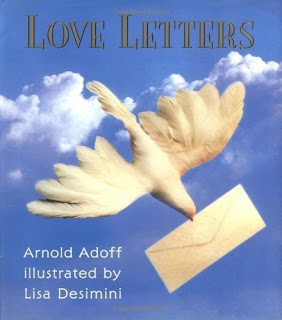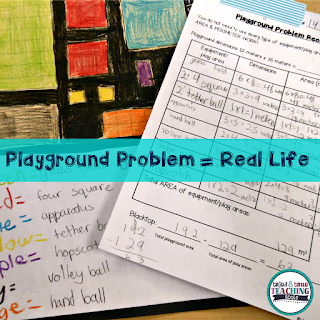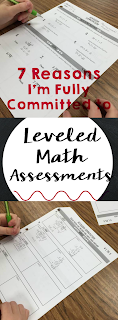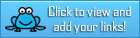# Category Archives : 100th Day

## Opinion Writing Peer Editing Checklist CCSS Aligned for Grades 3rd – 5th# Opinion Writing Peer Editing Checklist CCSS Aligned for Grades 3rd – 5th

The 4 peer editing checklists are very detailed (for a 3, 4, 5, & 7 paragraph essay) created for the progressing writer. Excellent for mixed or leveled classes to give students individualized instruction. Practical and easy to understand. Students will know what is expected of them and they will have opportunities to reflect on their work.

Included in this resource:
✓ How I Use This Resource
3, 4, 5, & 7 Paragraph:
✓ Peer Editing Checklist Half Page
✓ Peer Editing Checklist Full Page
✓ Graphics and Fonts Credit

Try part of the opinion essay bundle before you buy it right here…FREE Opinion Writing Basic Rubric CCSS Aligned

*Teacher rubric on one side while student self-assessment is on the other side when printed.
*Rubrics are created with variation to meet your classroom’s needs.
*Can print rubrics front and back to save on paper (cut it in half.)
*Peer edits for students to share and learn from each other.
*It’s a very detailed checklist.
*For a 3, 4, 5, & 7 paragraph opinion essay.
*Checklists are full & half page PDF format.
*Can print checklists front and back to save on paper.
*Practical and easy to understand rubrics for teachers and students.
*Easy scoring on a 10 or 5 points each category out of 100 points.
*Great for mixed or leveled classes to give students individualized instruction.
*Teacher and student self assessment to grade a 3, 4, 5, & 7 paragraph essay.
*Add CCSS.ELA-LITERACY.W.3-5.6 by having students research the Internet and type up the essay to implement technology for a cross-curricular assignment.
*Add CCSS.ELA-LITERACY.W.3-5.7 & W.3-5.8 by having students conduct a research project by assigning a prompt. Have them gather relevant information through printed or digital sources. Be sure to have them add a bibliography.
*Can be used as enrichment for 2nd grade students.

The way I use this resource…
In writing, I scaffold and model information to my students. Each class is different and each year is different; therefore, I can’t do everything the same each time I teach the same subject. I start with the basic rubric and introduce 1-3 new concepts each essay. Once I introduce a concept, I hold students accountable for the concepts I taught. If it appears that the majority of the class does not understand the concept, I do not hold them accountable and I reteach the concept. I try not to stifle their creativity by focusing on spelling, capitalization, and punctuation during the first two terms of school depending on the class. I also can easily use the 6 traits of writing with the rubrics. Make sure you choose a topic that is relevant, relatable, and the reasons are researchable.
Next, I introduce the peer editing checklist. I have students pair up and exchange papers. I have clipboards students can use while they sit around the room for this activity. It’s important for you to model for the students how to peer edit. Many times students just want to be nice and not give feedback. However, this is a great opportunity for students to read each others essays and learn from each other. I try not to let the same students peer edit with each other every time in order for students to view a variety of styles over the school year.
Finally, you are able to print front and back! Then you can cut them in half to save on paper. For example, pages 12 and 13 are the same rubric. Simply print it from your computer or printer as two-sided. If this does not work for you, print the first rubric for a full page rubric.
Happy Teaching!
Sally

SaveSave

## February Teacher Talk

Posted by Deann Marin of Socrates Lantern

Welcome to our February Teacher Talk.  All of us from the Teacher Talk collaborative would like wish you a Happy Valentines Day.  We have so many fab things this month from  proof-reading ideas, to ELA to math activities, to celebrating Black History Month and President’s Day, you don’t want to miss reading these blog posts from some awesome educators.If you’re interested in joining this unique group of teacher entrepreneurs and blogging buddies and our blog linky, sign up here….The Best of Teacher Entrepreneurs Marketing Cooperative. If you decide to join, be sure to mention one of our names.

<<<<<<<<<<<<<<<<<<<<<<<<<<<<<<<<<<<<<<<<<<<<<<<<<<<<<<<<<<<

The Proof is in the Pudding, Proof-Reading That Is!As a veteran English and Social Studies teacher, I’d like to share some effective proof-reading tips that I’ve used to help students improve their writing skills, and to make my life a bit easier.

<<<<<<<<<<<<<<<<<<<<<<<<<<<<<<<<<<<<<<<<<<<<<<<<<<<<<<<<<<<

Love is All We Need

By Retta London of Rainbow City LearningJust a little inspiration for building confidence and acceptance in your learning community.

<<<<<<<<<<<<<<<<<<<<<<<<<<<<<<<<<<<<<<<<<<<<<<<<<<<<<<<<<<<

IMWAYR: Funny Bones

By Lisa Robles of LisaTeachR’s ClassroomHave you read Funny Bones? Winner of multiple awards and a great informational addition to your Dia de Los Muertos collection!

<<<<<<<<<<<<<<<<<<<<<<<<<<<<<<<<<<<<<<<<<<<<<<<<<<<<<<<<<<<

Valentine’s Day STEM Olympics

By Kerry Tracy of Kerry TracyBlog post outlines 5 infatuating Valentine’s Day-themed STEM challenges that can be modified for use with grades 2-8. Help Cupid get some target practice, build a tower of love, design the perfect candy container, find the “heaviest” heart, and have a flower frenzy!

<<<<<<<<<<<<<<<<<<<<<<<<<<<<<<<<<<<<<<<<<<<<<<<<<<<<<<<<<<<

Cooperative Learning – Bring Core Subjects Together for Student Learning!

By M. Moore of Moore ResourcesCooperative Learning – Bring Core Subjects Together for Student Learning!

<<<<<<<<<<<<<<<<<<<<<<<<<<<<<<<<<<<<<<<<<<<<<<<<<<<<<<<<<<<

What Word Does This Say: B-L-E-N-D?

By Susan Berkowitz of Susan BerkowitzThis is part of a series of posts about phonological awareness and the different steps and skills to teach. This post is about blending sounds and syllables to hear the word.

<<<<<<<<<<<<<<<<<<<<<<<<<<<<<<<<<<<<<<<<<<<<<<<<<<<<<<<<<<<

Teaching Self-Regulation Skills to Elementary Age Children

By Thia Triggs of Print PathFinally! A systematic method to teach self-regulation skills to children with sensory, emotional, and behavioral needs. Color coded task cards give students managed choices to learn specific skills that meet their sensory needs and also calm them down, so that they can perform their academic classroom tasks.

<<<<<<<<<<<<<<<<<<<<<<<<<<<<<<<<<<<<<<<<<<<<<<<<<<<<<<<<<<<

My Funny Valentine: Love Letters by Arnold Adoff

By Tracy Willis of Wild Child DesignsA review and glimpse into “Love Letters” poetry by Arnold Adoff. This post also includes a teaching idea for its use in the classroom.

<<<<<<<<<<<<<<<<<<<<<<<<<<<<<<<<<<<<<<<<<<<<<<<<<<<<<<<<<<<

Robots + Math & Science = Total Engagement

By Megan Bodmann of Adventures Teaching 4thGet your students engaged in learning by introducing robots into your classroom. They are not only a ton of fun, but you can easily utilize them in your math and science lessons. Find out how easy it is to do!

<<<<<<<<<<<<<<<<<<<<<<<<<<<<<<<<<<<<<<<<<<<<<<<<<<<<<<<<<<<

Playground Problem = Real Life

By Kathie Yonemura of Tried and True Teaching ToolsThe Playground Problem is a real-life math challenge! It keep students engaged and practicing their area and perimeter skills in a meaningful way.

<<<<<<<<<<<<<<<<<<<<<<<<<<<<<<<<<<<<<<<<<<<<<<<<<<<<<<<<<<<

Understanding Fractions: A 6-Part Series

By Shametria Routt of The Routty Math TeacherFractions– a single word that deflates the confidence of our most competent students and adults alike. In this series, I share some of the essential fraction understandings that I have developed over the years, including the many math tools you can use to reinforce these essential skills. With that in mind, each of the six posts highlights a fraction tool and activity that can be used to address a specific Common Core Math Standard (with a few printable freebies too).

<<<<<<<<<<<<<<<<<<<<<<<<<<<<<<<<<<<<<<<<<<<<<<<<<<<<<<<<<<<

Leveled Math Assessments

By Tammy Roose of Tarheel State TeacherDo your math assessments encourage a growth mindset? Create an entry point for students who are not yet meeting the standards? Allow above average learners to show they know more than just what’s expected for their grade-level? Find out 7 reasons why I’m committed to leveled math assessments this year!

<<<<<<<<<<<<<<<<<<<<<<<<<<<<<<<<<<<<<<<<<<<<<<<<<<<<<<<<<<<

Here’s your chance to hop on over and visit the blog posts of our creative teachers.## The Best of Teacher Entrepreneurs Marketing Cooperative – Categories

Hi everyone,

Thanks for stopping by our Word Press blog, The Best of Teacher Entrepreneurs Marketing Cooperative.  Click on the categories posted on the right hand column to find particular lessons. Below is a list of the categories.

Have fun exploring TBOTEMC,

Vicky

TBOTEMC CATEGORIES:

CLASSROOM MANAGEMENT

CLIP ART

COMMON CORE

FEATURED SELLERS

FREE LESSONS

FREE LESSONS & PRICED LESSONS – The Best of Teacher Entrepreneurs

• PreKindergarten
• Kindergarten
• College

HOLIDAYS

• 100th Day
• 4th of July
• Christmas
• Easter
• End of the Year
• Father’s Day
• Flag Day
• Halloween
• Martin Luther King, Jr.
• Memorial Day
• Mother’s Day
• New Year’s Day
• Presidents’ Day
• St. Patrick’s Day
• Thanksgiving
• Valentine’s Day
• Veteran’s Day

HOME

LANGUAGE ARTS LESSONS

MATH LESSONS

MISC. LESSONS

SCIENCE LESSONS

SOCIAL STUDIES LESSONS

SPECIAL EDUCATION

TEST PREPARATION

UNCATEGORIZED